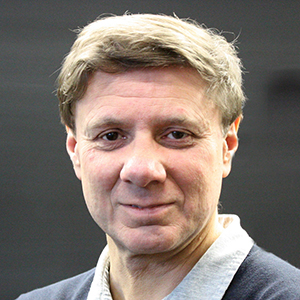Martin Barlow

Martin Barlow is Emeritus Professor of Mathematics at the University of British Columbia. He received his BA from Cambridge in 1975, and his PhD from the University of Wales in 1979. Prior to his move to UBC in 1992, he held postdoctoral and faculty positions at Liverpool University, Trinity College Cambridge, and the University of Cambridge. In 1998 he was elected as a Fellow of the Royal Society of Canada, and in 2006 of the [UK] Royal Society. Martin’s main research contributions have been on random walks and diffusions. In 1988, with Ed Perkins, he wrote one of the founding papers in the field of diffusions on fractals, on Brownian motion on the Sierpinski gasket. Subsequent research with Richard Bass developed more general techniques to study processes of this kind. Following his 2004 paper on random walks on supercritical percolation clusters, his research has extended on random walks on random graphs.

Random walks on graphs with fractal structure

This series of talks will study random walks on graphs with irregular, random or fractal structure. The motivation goes back to a 1976 article by the physicist Pierre de Gennes on percolation in La Recherche. He discussed the wide applicability of this model, and suggested that our understanding of percolation clusters would be assisted by looking at random walks on these objects: he called these random walks “the ant in the labyrinth.” It was conjectured in 1976, and has been proved in a number of cases since, that critical models in statistical physics have fractal properties. For example, for such a graph embedded in d-dimensional Euclidean space, one may find that the number of points within a graph distance R of the origin grows as R α, with α < d. If this happens, α can be called the “fractal dimension” of the graph.

Wald lecture I. Random walks and fractal graphs

This lecture will begin with a review of percolation, and of the questions raised by de Gennes in 1976. Since random fractals are hard, initial research in this area looked at deterministic exact fractals, and the graphs that can be associated naturally with them. Of these, the simplest is the Sierpinski gasket (SG), and I will review the properties of random walk on the associated infinite graph. Similar properties hold for some other families of graphs. Most of the initial work on these spaces used direct probabilistic methods, often very specific to the particular space. The search for a more robust theory leads one to look for more flexible tools: the first is given by the connection between random walks and electrical networks noted by Doyle and Snell; I will conclude with a review of this.

Wald lecture II. Low dimensional random fractals

In many circumstances, the behaviour of the random walk and its transition probabilities can be described by two indices, called by physicists the “fractal” and “walk” dimensions, and denoted by df and dw. This lecture will look at the tools that enable us to calculate these and obtain the associated transition probability or heat kernel bounds. Pioneering work by Grigoryan and Telcs established that three kinds of estimate are needed:  control of the size of balls,  control of the resistance across annuli, and  a smoothness result (a Harnack inequality). In the “low-dimensional case” the Harnack inequality is not needed, and  can be replaced by easier bounds on the resistance between points. Fortunately, it turns out that many random fractals of interest in statistical physics are low-dimensional, and one can therefore calculate random walk indices. Examples are critical branching processes, the incipient infinite cluster (IIC) for percolation in high dimensions, and the uniform spanning tree in dimensions d=2 and d≥5. The IIC in d=2 remains a challenge: while SLE allows one to calculate many indices associated with critical percolation in two dimensions, it does not provide enough information to give us df or dw.

Wald lecture III. Higher dimensional spaces

The final lecture will look spaces where the low dimensional methods do not apply. I will begin by discussing supercritical percolation clusters, where the random walk has Gaussian-type bounds, and also satisfies an invariance principle. I will then discuss the challenge posed by fractal graphs with high dimension. I will conclude by reviewing models in the plane, such as the uniform infinite planar triangulation, a topic with connections to the Gaussian free field and Liouville quantum gravity.